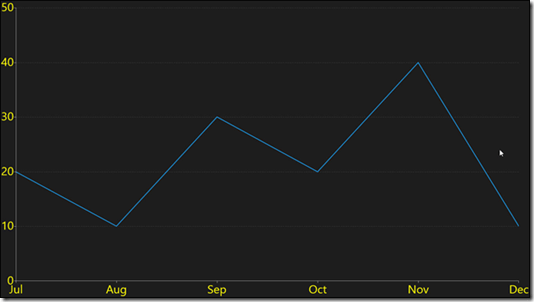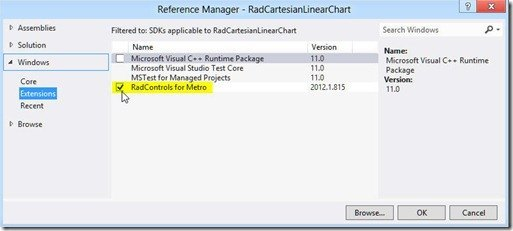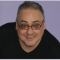In this post, I will explore creating a line chart using the RadCartesianChart from the Telerik Windows 8 UI Controls.  You can Download Telerik Widows 8 Controls here.

Figure 1 shows the chart we wish to build. This is a linear chart built with a RadCartesianChart. It represents revenue for the last six months of 2012,The best way to approach building a line chart (or any chart) is to start with the data you wish to display. In this case, we will use mock data representing Monthly Revenue earned from July through December 2012. The class that will hold our data is MonthRevenue.cs,

``public` `class` `MonthRevenue``{``    ``public` `DateTime Date { ``get``; ``set``; }``    ``public` `double` `Amount { ``get``; ``set``; }``}` `

The chart itself will be bound using a ViewModel class (as part of the Model/View/ViewModel architecture). The VM in this case is fairly straight forward except that it must also do the work of generating our “dummy” data,

``public` `class` `MainViewModel : INotifyPropertyChanged``{``    ``public` `MainViewModel()``    ``{``        ``LoadData();``    ``}` `    ``private` `void` `LoadData()``    ``{``        ``var myRevenues = ``new` `List<MonthRevenue>();``        ``myRevenues.Add( ``new` `MonthRevenue() ``              ``{ Amount = 20, Date = ``new` `DateTime( 2012, 7, 10 ) } );``        ``myRevenues.Add( ``new` `MonthRevenue() ``              ``{ Amount = 10, Date = ``new` `DateTime( 2012, 8, 10 ) } );``        ``myRevenues.Add( ``new` `MonthRevenue() ``              ``{ Amount = 30, Date = ``new` `DateTime( 2012, 9, 10 ) } );``        ``myRevenues.Add( ``new` `MonthRevenue() ``              ``{ Amount = 20, Date = ``new` `DateTime( 2012, 10, 10 ) } );``        ``myRevenues.Add( ``new` `MonthRevenue() ``              ``{ Amount = 40, Date = ``new` `DateTime( 2012, 11, 10 ) } );``        ``myRevenues.Add( ``new` `MonthRevenue() ``              ``{ Amount = 10, Date = ``new` `DateTime( 2012, 12, 10 ) } );``        ``Revenues = myRevenues;``    ``}` `    ``private` `List<MonthRevenue> revenues;``    ``public` `List<MonthRevenue> Revenues``    ``{``        ``get` `{ ``return` `revenues; }``        ``set``        ``{``            ``revenues = value;``            ``OnPropertyChanged();``        ``}``    ``}` `    ``public` `event` `PropertyChangedEventHandler PropertyChanged;``    ``private` `void` `OnPropertyChanged(``               ``[CallerMemberName] ``string` `caller = ``""` `)``    ``{``        ``if` `( PropertyChanged != ``null` `)``        ``{``            ``PropertyChanged( ``this``, ``new` `PropertyChangedEventArgs( caller ) );``        ``}``    ``}` `}` `

Notice that each entry in the myRevenues collection is of type MonthRevenue, and thus has an amount and a Date (the DateTime is created in the order year, month, date).

With the data ready, we can now create our chart.Next, open MainPage.xaml and add a using statement for the Telerik controls

` `xmlns:telerik="using:Telerik.UI.Xaml.Controls"``

We’ll be using a style to set the labels both on the x and y axis (as seen in the figure above) so let’s create that as a resource,

``<``Page.Resources``>``     ``<``Style``         ``x:Key``=``"LabelStyle"``         ``TargetType``=``"TextBlock"``>``         ``<``Setter``             ``Property``=``"FontSize"``             ``Value``=``"30"``></``Setter``>``         ``<``Setter``             ``Property``=``"Foreground"``             ``Value``=``"Yellow"``></``Setter``>``     ``</``Style``>`` ``</``Page.Resources``>` `

``<``Grid``    ``Background``=``"{StaticResource ApplicationPageBackgroundThemeBrush}"``>``    ``<``telerik:RadCartesianChart``        ``Name``=``"xRadChart"``>``

Within the chart we’ll declare a Grid, and within that we’ll set that we want our Major Lines to be visible,

``<``telerik:RadCartesianChart.Grid``>``    ``<``telerik:CartesianChartGrid``        ``MajorLinesVisibility``=``"Y"``/>``</``telerik:RadCartesianChart.Grid``>``

Next, we’ll set the Horizontal and vertical axes. The HorizontalAxis is slightly more complicated because not only do we want it to be categorical, but we want a special type of Categorical axis, we want a DateTime that is going to mark the months,

``<``telerik:RadCartesianChart.HorizontalAxis``>``    ``<``telerik:DateTimeCategoricalAxis``        ``DateTimeComponent``=``"Month"``        ``LabelFormat``=``"{}{0,0:MMM}"``        ``LabelStyle``=``"{StaticResource LabelStyle}"` `/>`` ``</``telerik:RadCartesianChart.HorizontalAxis``>` `

The vertical axis is more straightforward but note that it uses the same labelstyle.

``<``telerik:RadCartesianChart.VerticalAxis``>``    ``<``telerik:LinearAxis`  `LabelStyle``=``"{StaticResource LabelStyle}"``/>``</``telerik:RadCartesianChart.VerticalAxis``>``

We’re now ready to add the Line series, binding its value to the Revenues property of our VM. We need to specify the Value and the Category binding so that the chart knows which data to retrieve for each axis.

``<``telerik:LineSeries``     ``ItemsSource``=``"{Binding Revenues}"``>``     ``<``telerik:LineSeries.ValueBinding``>``         ``<``telerik:PropertyNameDataPointBinding``             ``PropertyName``=``"Amount"` `/>``     ``</``telerik:LineSeries.ValueBinding``>``     ``<``telerik:LineSeries.CategoryBinding``>``         ``<``telerik:PropertyNameDataPointBinding``             ``PropertyName``=``"Date"` `/>``     ``</``telerik:LineSeries.CategoryBinding``>`` ``</``telerik:LineSeries``>` `

There are a number of ways to set the VM as the DataContext for the chart, we’ll do so in the OnNavigatedTo event handler in the code behind,

``protected` `override` `void` `OnNavigatedTo(NavigationEventArgs e)`` ``{``     ``xRadChart.DataContext =``new` `MainViewModel();`` ``}` `

That’s it, you now have a fully functioning chart displaying revenues over months.

Source code for this example can be found here.